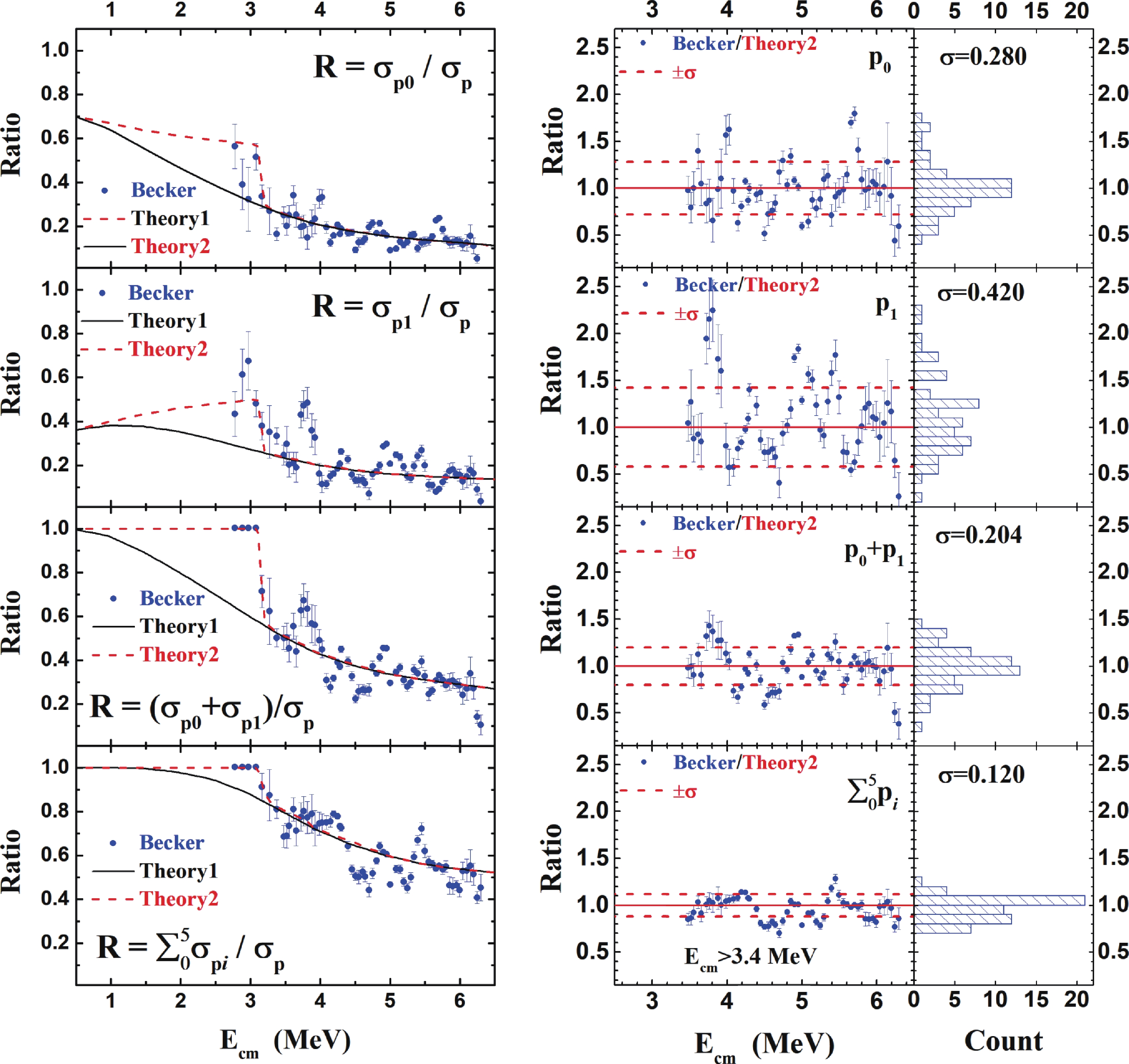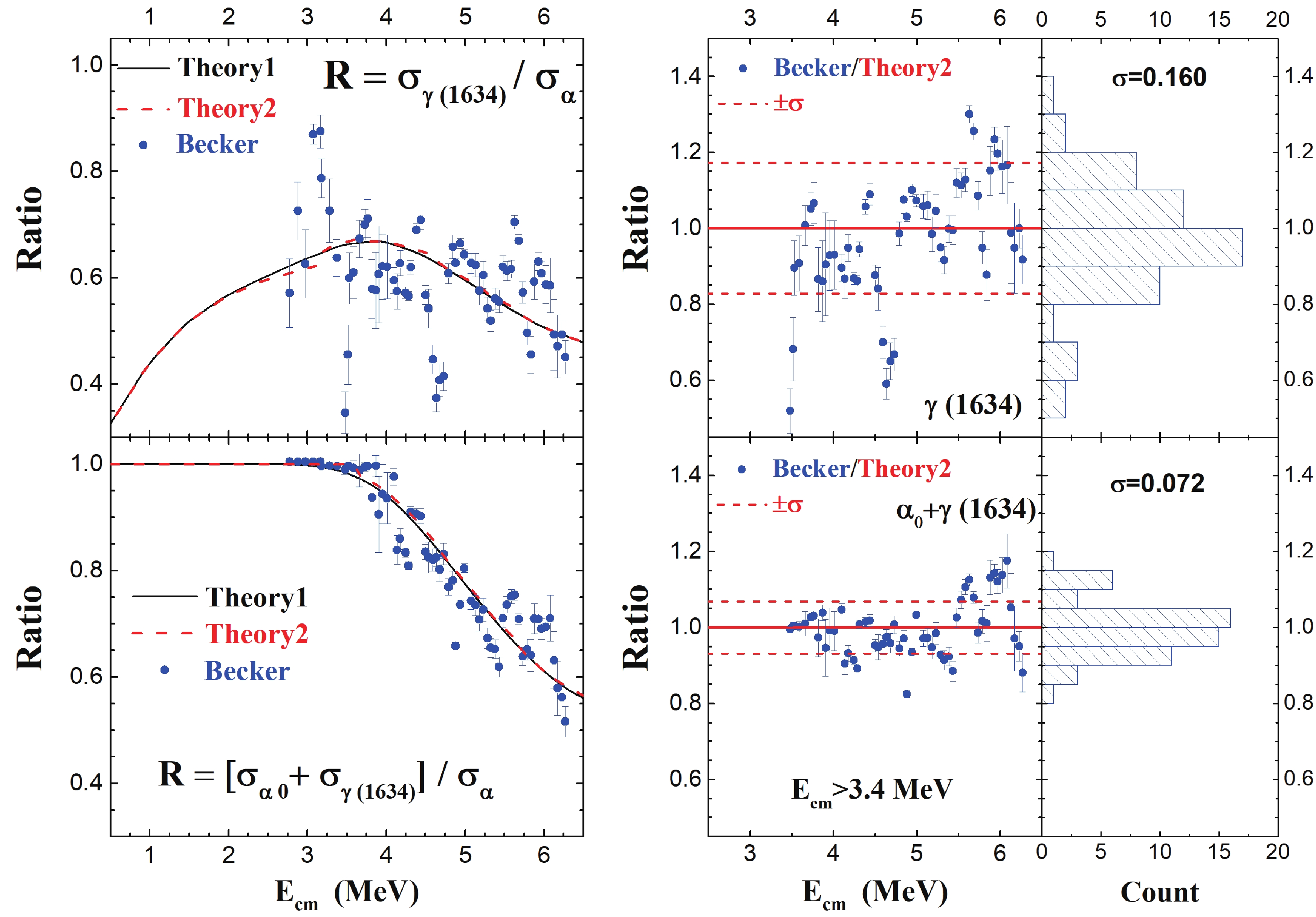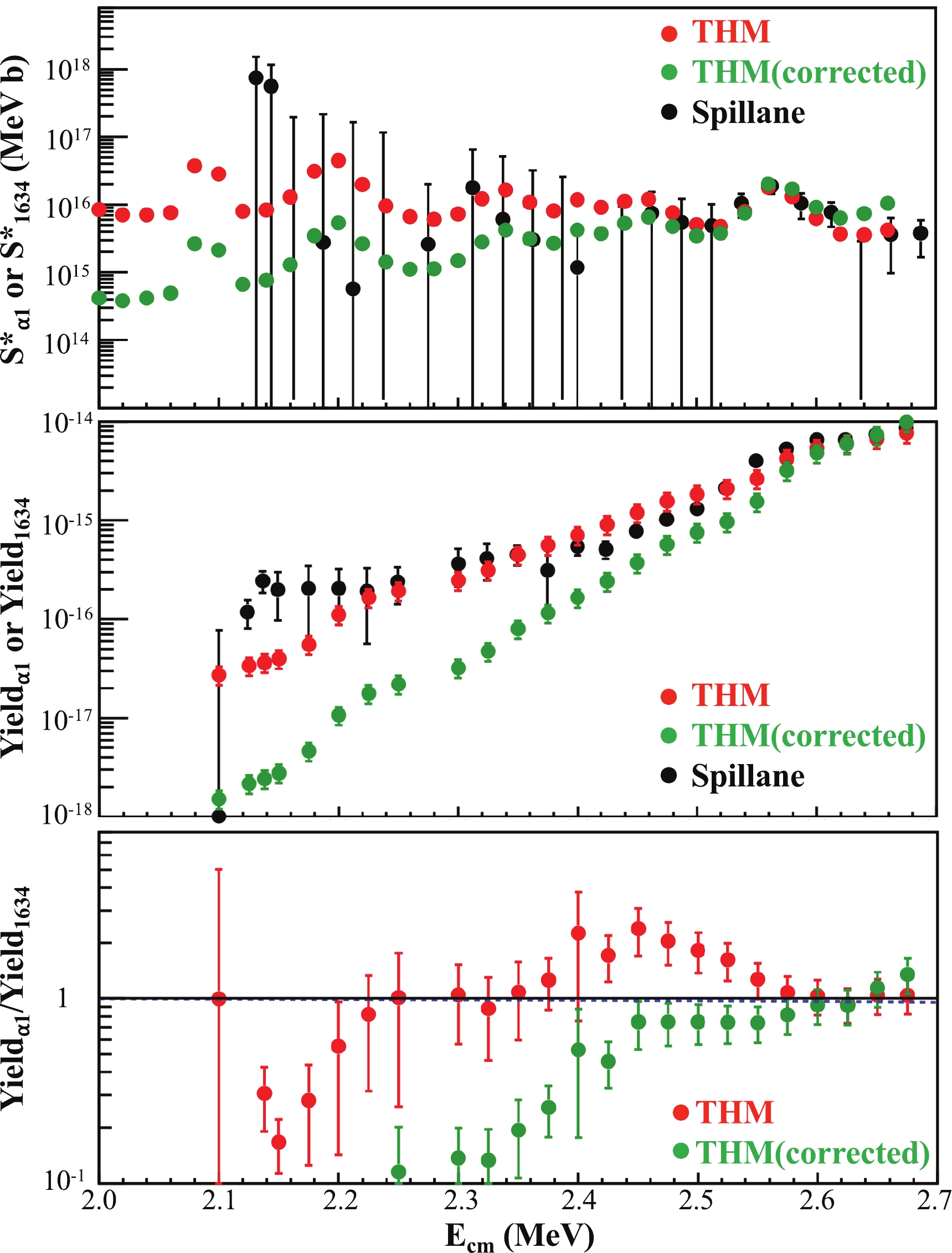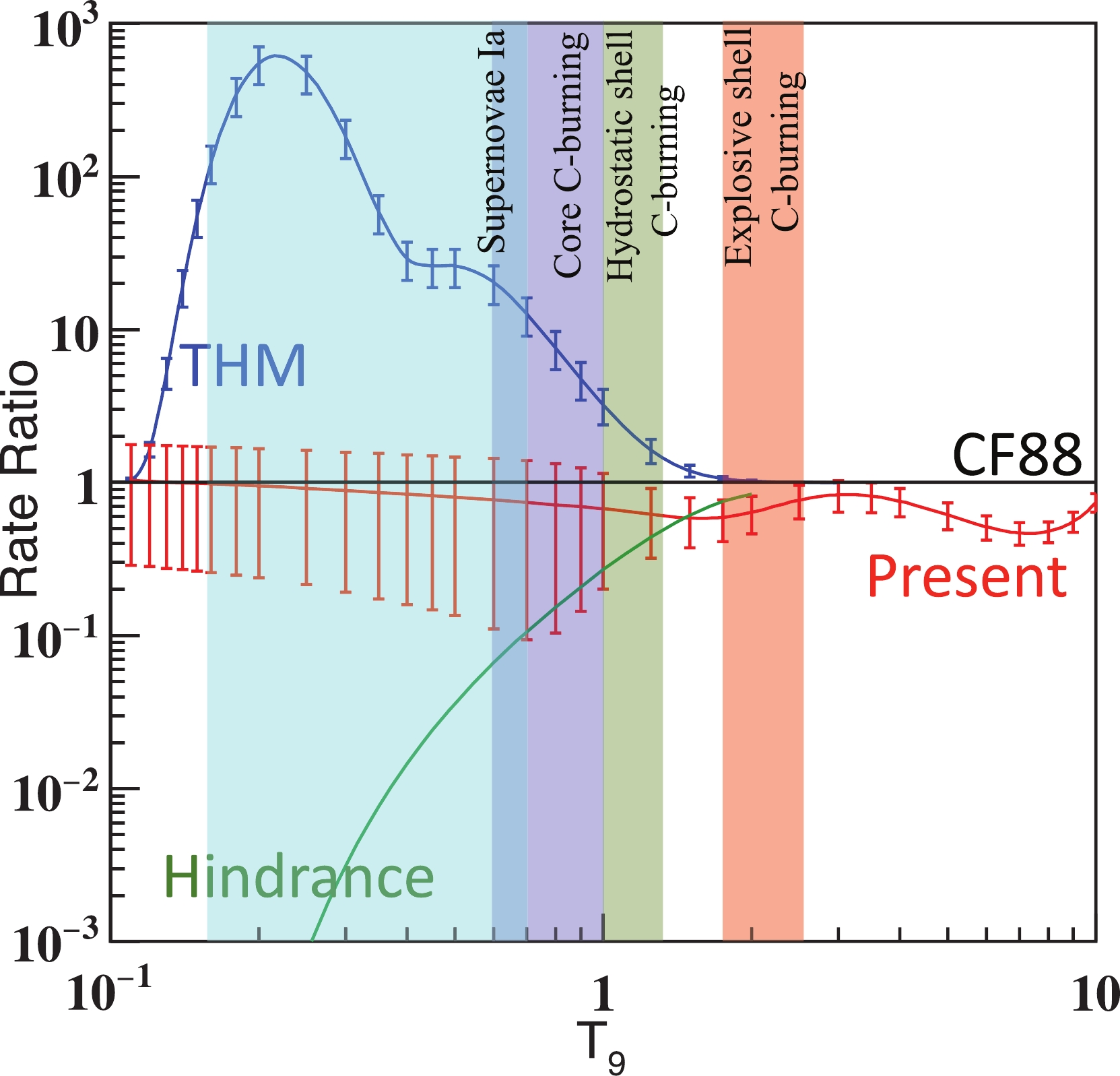Modified astrophysical S-factor of 12C+12C fusion reaction at sub-barrier energies

• The 12C+12C fusion reaction plays a crucial role in stellar evolution and explosions. Its main open reaction channels include $\alpha$, p, n, and 8Be. Despite more than a half century of efforts, large differences remain among the experimental data of this reaction measured using various techniques. In this work, we analyze the existing data using a statistical model. Our calculation shows the following: 1) the relative systematic uncertainties of the predicted branching ratios decrease as the predicted ratios increase; 2) the total modified astrophysical S-factors (S* factors) of the p and $\alpha$channels can be obtained by summing the S* factors of their corresponding ground-state transitions and the characteristic $\gamma$rays, while taking into account the contributions of the missing channels to the latter. After applying corrections based on branching ratios predicted by the statistical model, an agreement is achieved among the different data sets at Ecm > 4 MeV, while some discrepancies remain at lower energies, suggesting the need for better measurements in the near future. We find that the S* factor recently obtained from an indirect measurement is inconsistent with the direct measurement value at energies below 2.6 MeV. We recommend upper and lower limits for the 12C+12C S* factor based on the existing models. A new 12C+12C reaction rate is also recommended.
••Get Citation
Y. J. Li, X. Fang, B. Bucher, K. A. Li, L. H. Ru and X. D. Tang. The modified astrophysical S-factor of the 12C+12C fusion reaction at sub-barrier energies[J]. Chinese Physics C. doi: 10.1088/1674-1137/abae56
Y. J. Li, X. Fang, B. Bucher, K. A. Li, L. H. Ru and X. D. Tang. The modified astrophysical S-factor of the 12C+12C fusion reaction at sub-barrier energies[J]. Chinese Physics C.Milestone
Article Metric

Article Views(536)
Cited by(0)
Policy on re-use
To reuse of subscription content published by CPC, the users need to request permission from CPC, unless the content was published under an Open Access license which automatically permits that type of reuse.
通讯作者: 陈斌, bchen63@163.com
• 1.

沈阳化工大学材料科学与工程学院 沈阳 110142

Title:
Email:

Modified astrophysical S-factor of 12C+12C fusion reaction at sub-barrier energies

Corresponding author: X. Fang, fangx26@mail.sysu.edu.cn
• 1. China Institute of Atomic Energy, Beijing 102413, China
• 2. Sino-French Institute of Nuclear Engineering and Technology, Sun Yat-sen University, Zhuhai 519082, China
• 3. Idaho National Laboratory, Idaho Falls, ID 83415, USA
• 4. Institute of Modern Physics, Chinese Academy of Sciences, Lanzhou 730000, China
• 5. School of Nuclear Science and Technology, University of Chinese Academy of Sciences, Beijing 100049, China

Abstract: The 12C+12C fusion reaction plays a crucial role in stellar evolution and explosions. Its main open reaction channels include $\alpha$, p, n, and 8Be. Despite more than a half century of efforts, large differences remain among the experimental data of this reaction measured using various techniques. In this work, we analyze the existing data using a statistical model. Our calculation shows the following: 1) the relative systematic uncertainties of the predicted branching ratios decrease as the predicted ratios increase; 2) the total modified astrophysical S-factors (S* factors) of the p and $\alpha$channels can be obtained by summing the S* factors of their corresponding ground-state transitions and the characteristic $\gamma$rays, while taking into account the contributions of the missing channels to the latter. After applying corrections based on branching ratios predicted by the statistical model, an agreement is achieved among the different data sets at Ecm > 4 MeV, while some discrepancies remain at lower energies, suggesting the need for better measurements in the near future. We find that the S* factor recently obtained from an indirect measurement is inconsistent with the direct measurement value at energies below 2.6 MeV. We recommend upper and lower limits for the 12C+12C S* factor based on the existing models. A new 12C+12C reaction rate is also recommended.

HTML1.   Introduction2.   Statistical model calculation3.   Comparisons between calculations and experimental results4.   Connection between particle spectroscopy and $\gamma$-ray spectroscopy5.   S* factors of 12C(12C,p)23Na and 12C(12C,$\alpha$)20Ne at Ecm >2.7 MeV6.   Comparison of indirect and direct measurements at Ecm$<$ 2.7 MeV7.   Extrapolation of total 12C+12C S* factor8.   12C+12C reaction rate
Reference (51)
PDF查看关注分享

Top

目录/DownLoad:  Full-Size Img  PowerPoint